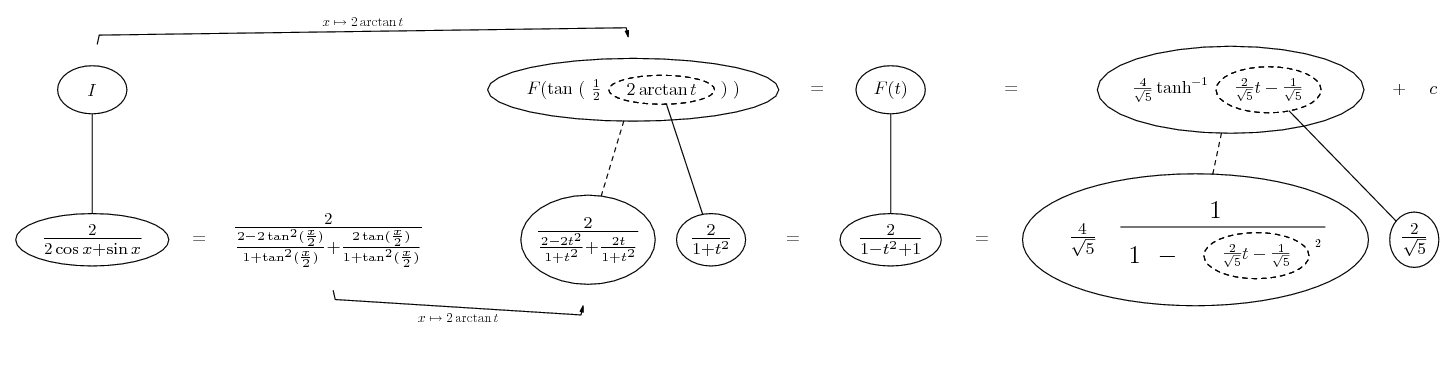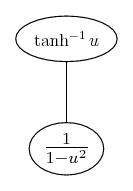# Thread: Integration by substitution

1. ## Integration by substitution

I think that Integration by substitution is the appropriate method to use for the following function.

$\displaystyle \displaystyle\int\dfrac{2\sec x}{2+\tan x}$

I can simplify it to

$\displaystyle \displaystyle\int\dfrac{2}{2\cos x + \sin x}$

But I don't know if this is any better. Any ideas please

Thanks

2. You could try rewriting it as:

$\displaystyle \frac{2sec(x)}{2+tan(x)}=\frac{2}{\sqrt{5}cos(x-tan^{-1}(\frac{1}{2}))}$

3. Hi

Could you explain how you got the simplification please.

James

4. You had $\displaystyle \frac{2}{2cos(x)+sin(x)}$. Which is cool.

$\displaystyle \frac{2}{\sqrt{5}(\frac{2cos(x)}{\sqrt{5}}+\frac{s in(x)}{\sqrt{5}})}$

Now, note that $\displaystyle cos(x-tan^{-1}(1/2))$
$\displaystyle =cos(x)cos(tan^{-1}(1/2))+sin(x)sin(tan^{-1}(1/2))=\frac{2cos(x)}{\sqrt{5}}+\frac{sin(x)}{\sqrt{5 }}$

Just a matter of some algebra and noting an identity or two.

5. Thanks, I see where your coming from now.

Sorry to ask another question but how should I handle the substitution?

James

6.Originally Posted by bobredI think that Integration by substitution is the appropriate method to use for the following function.

$\displaystyle \displaystyle\int\dfrac{2\sec x}{2+\tan x} \, {\color{red}dx}$

I can simplify it to

$\displaystyle \displaystyle\int\dfrac{2}{2\cos x + \sin x} \, {\color{red}dx}$

But I don't know if this is any better. Any ideas please

Thanks
Use the weierstrass substitution: The Weierstrass Substitution Example

7. Thanks Mr Fantastic for the reply, I've tried to follow the example several times to no avail, any tips would be appreciated.

Thanks

8. Just in case a picture helps...

Spoiler:Straight continuous lines differentiate downwards, the straight dashed line likewise but with respect to the dashed balloon expression. So the triangular network on the right satifies the chain rule. The Weierstrass substitution is 't = tan of half the angle', from which are derived the formulae for sin x and cos x in terms of t.

Don't integrate - balloontegrate!

Balloon Calculus Forum

9. Hi

Thanks for the responce, I feel so thick. How did you arrive at

10. Well, anti-clockwise from bottom-left, we do the Weierstrass subs for cos and sin (Mr Fantastic's link), simplify, then spot that

$\displaystyle \frac{2}{1 - t^2 + t}$

is like the bottom end of the standard result for tanh-1...So complete the square...

11. ... and the resemblance...

$\displaystyle 1 - t^2 + t$

$\displaystyle = 1 - \large{[}(t - \frac{1}{2})^2 - \frac{1}{4}\large{]}$

$\displaystyle = \frac{5}{4} - (t - \frac{1}{2})^2$

$\displaystyle = \frac{5}{4} [1 - \frac{4}{5}(t - \frac{1}{2})^2]$

$\displaystyle = \frac{5}{4} [1 - (\frac{2}{\sqrt5}t - \frac{1}{\sqrt5})^2]$

Don't forget you still have to revert from t to x.

12. Hi

Got it, thanks

#### Search Tags

integration, substitution